# Prime Numbers

Go back to  'Numbers'

We know that prime numbers are special, but what makes them so unique? This video will give you a little teaser before you become a prime number champion.

 1 Understanding Prime Numbers 2 What are Prime Numbers? 3 List of Prime Numbers between 1 to 100 4 Prime Numbers Chart 5 Largest Prime Number 6 Twin Prime Numbers 7 Coprime Numbers 8 How to find Prime Numbers? 9 Solved Examples on Prime Numbers 10 Common Mistakes 11 Prime Numbers Calculator 12 Thinking out of the Box! 13 Practice Questions on Prime Numbers 14 Important Topics of Prime Numbers 15 Maths Olympiad Sample Papers 16 Frequently Asked Questions (FAQs)

We at Cuemath believe that Math is a life skill. Our Math Experts focus on the “Why” behind the “What.” Students can explore from a huge range of interactive worksheets, visuals, simulations, practice tests, and more to understand a concept in depth.

Book a FREE trial class today! and experience Cuemath’s LIVE Online Class with your child.

## Understanding Prime Numbers

If a number can be divided into equal groups, then it is not a Prime number. It is a Composite number.

If a number cannot be divided into equal groups, then it is a Prime number.

Keep in mind:

• groups cannot be of size one.
• groups cannot have fractional quantities like $$\frac{1}{2}$$ in each group.

Do you think that you can divide things into groups with an equal number of things?

For example, we can divide $$12$$ circles into groups with an equal number of circles in different ways: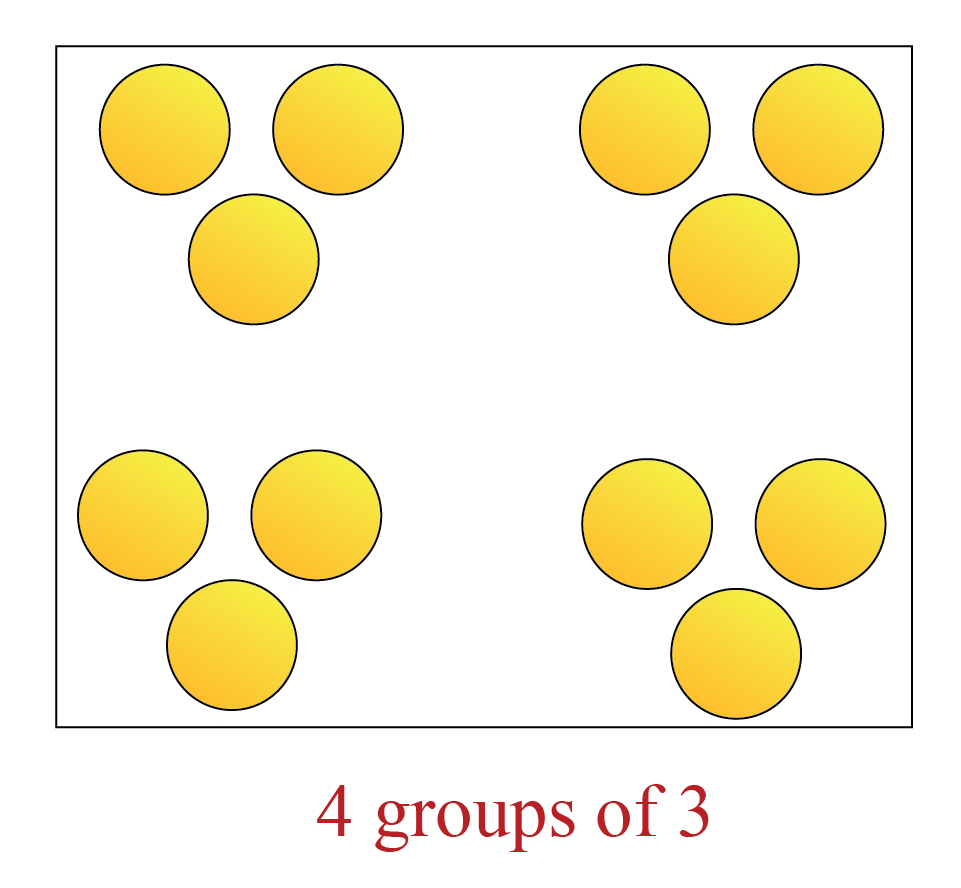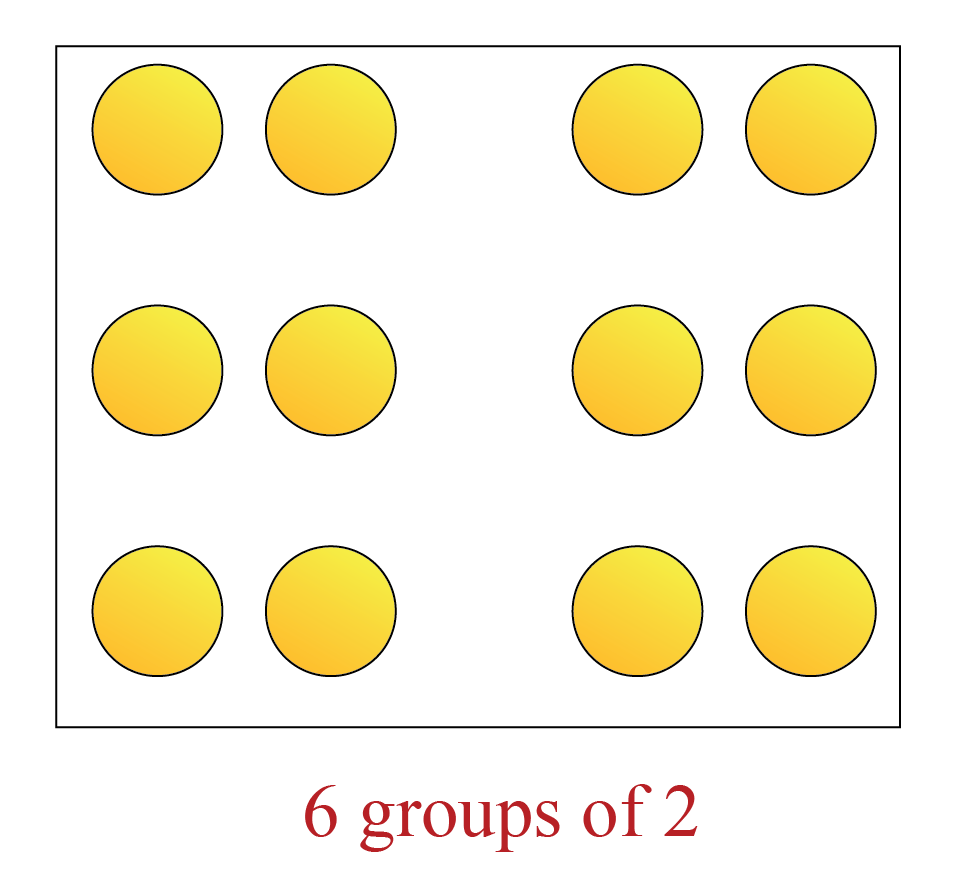This is because $$12$$ can be factorized in the following ways:

• $$12 = 4 \times 3$$
• $$12 = 6 \times 2$$

We know that $$12$$ can be factorized in the following ways as well:

• $$3$$ groups of $$4$$ because $$3 \times 4 =12$$
• $$2$$ groups of $$6$$ because $$2 \times 6 =12$$

(1 group of 12 or 12 groups of 1 don't count in our current system)

Quick recall: $$1, 2, 3, 4, 6$$ and $$12$$ are called factors of $$12$$.

So what we understood from the above example is that we can divide a number into groups with equal numbers of items/elements only if it can be factorized as the product of two numbers.

But it is not possible to divide all numbers into groups with equal numbers.

For example, $$7$$ cannot be divided into groups of equal numbers.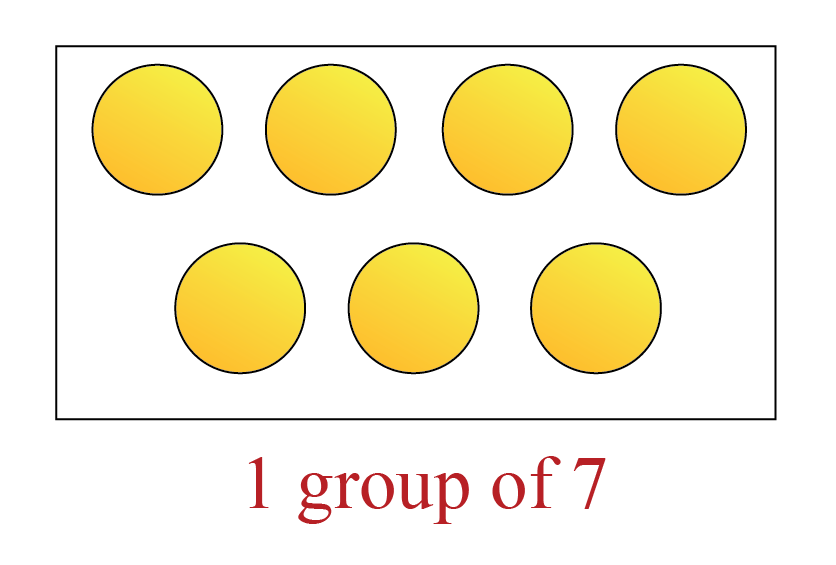This is because $$7$$ can only be factorized as follows:

• $$7 \times 1 = 7$$
• $$1 \times 7 = 7$$

Quick recall: $$1$$ and $$7$$ are the only factors of $$7$$.

In the above examples:

• $$12$$ is NOT a prime number (it means it is a composite number) because it could be divided into groups of equal numbers).
• $$7$$ is a prime number because it could NOT be divided into groups of equal numbers.

## What are Prime Numbers?

Any whole number greater than $$1$$ that has exactly two factors, $$1$$ and itself, is defined as a prime number.

Another equivalent definition is:

Any whole number greater than $$1$$ that is divisible only by $$1$$ and itself, is defined as a prime number.

Any number greater than 1 that is not a prime number, is defined to be a composite number. Thus composite numbers will always have more than 2 factors.

Any whole number greater than 1 is either a prime or composite number.

For example:

• The number $$15$$ has more than two factors: $$1, 3, 5,$$ and $$15$$.
Hence it is a composite number.
• On the other hand, $$13$$ has just two factors: $$1$$ and $$13$$.
Hence it is a prime number.

### Is 1 a Prime Number?

• 1 is neither prime nor composite, as the definition of prime numbers talks only about the whole numbers that are greater than $$1$$.
• $$1$$ has only one factor. So it cannot be a prime number as a prime number should have exactly two factors.
• $$1$$ has only one factor. So it cannot be a composite number as a composite number should have more than two factors.

### Characteristics of Prime Numbers

The characteristics of prime numbers are:

• A prime number is a whole number greater than $$1$$.
• A prime number has exactly two factors, i.e. $$1$$ and itself.

### Prime and Composite Numbers

A prime number is a whole number greater than $$1$$ that has EXACTLY two factors.

Example:

$$5$$ can be factorized in only one way. i.e., $$1 \times 5$$ (OR) $$5 \times 1$$.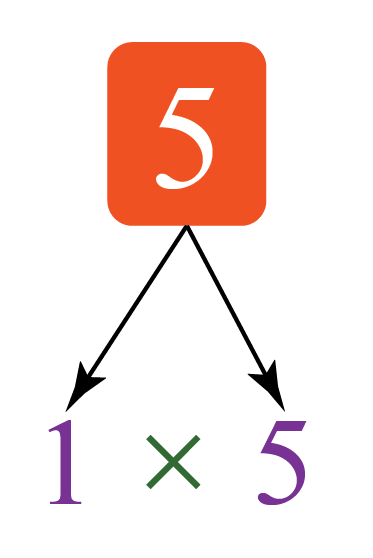It has only two factors $$5$$ and $$1$$.

So $$5$$ is a prime number.

A composite number is a whole number greater than $$1$$ that has MORE THAN two factors.

Example:

$$4$$ can be factorized in multiple ways.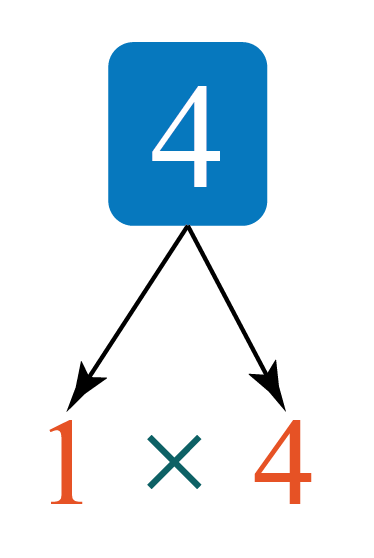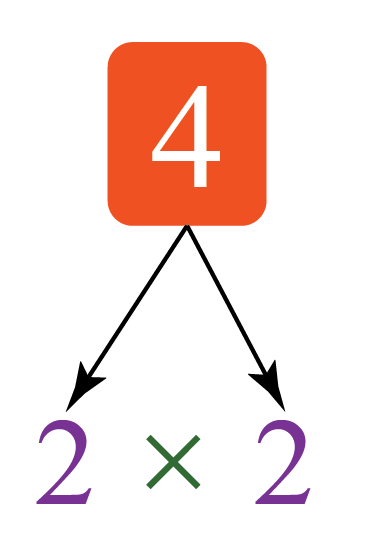So the factors of $$4$$ are $$1, 2 \text{ and } 4$$. It has more than two factors.

So $$4$$ is a composite number.

CLUEless in Math? Check out how CUEMATH Teachers will explain Prime Numbers to your kid using interactive simulations & worksheets so they never have to memorise anything in Math again!

Explore Cuemath Live, Interactive & Personalised Online Classes to make your kid a Math Expert. Book a FREE trial class today!

## List of Prime Numbers Between 1 to 100

There are $$25$$ prime numbers from $$1$$ to $$100$$.

The prime numbers between $$1$$ to $$100$$ are:

2, 3, 5, 7, 11, 13, 17, 19, 23, 29, 31, 37, 41, 43, 47, 53, 59, 61, 67, 71, 73, 79, 83, 89, 97

These numbers are listed in the following table:

 Prime numbers between 1 to 10 $$2, 3, 5, 7$$ Prime numbers between 11 to 20 $$11, 13, 17, 19$$ Prime numbers between 21 to 30 $$23, 29$$ Prime numbers between 31 to 40 $$31, 37$$ Prime numbers between 41 to 50 $$41, 43, 47$$ Prime numbers between 51 to 100 $$53, 59, 61, 67, 71,$$ $$73, 79, 83, 89, 97$$

## Prime Numbers Chart

Here is the prime numbers chart from $$1$$ to $$100$$.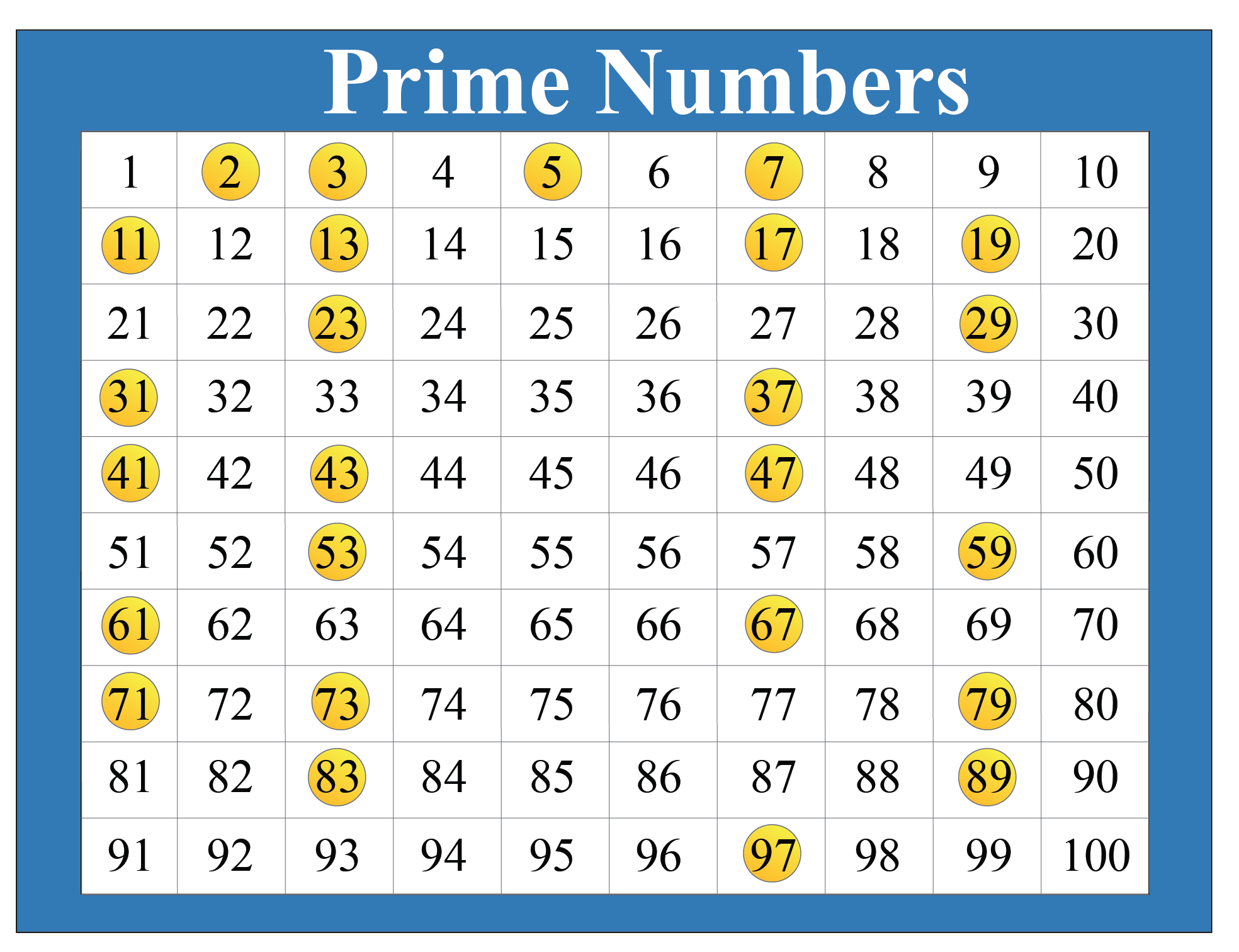Here is how we can find the list of prime numbers between $$1$$ to $$100$$.

We just strike off 1 as it is NOT a prime number. Then we strike off all the multiples of other numbers.

Then, the remaining numbers are the prime numbers.

## Largest Prime Number

We cannot determine the largest prime number.

The largest known prime number is $$2^{82,589,933}$$-1 having $$24,862,048$$ digits.

## Twin Prime Numbers

A pair of prime numbers that differ by $$2$$ are called twin prime numbers.

i.e. two prime numbers with a composite number in between are called twin primes.

For example:

1.  $$3$$ and  $$5$$ are twin primes.
2.  $$5$$ and  $$7$$ are twin primes.
3.  $$11$$ and  $$13$$ is another pair of twin primes.

## Coprime Numbers

If a pair of numbers has no common factor apart from $$1$$, then they are called coprime numbers

Example 1:

$$5$$ and $$9$$ are co-primes. That’s because:

Factors of $$5$$ are $$1$$ and $$5$$.

Factors of $$9$$ are $$1, 3$$ and $$9$$.

Here $$5$$ and $$9$$ have no common factors apart from $$1$$, making them coprime.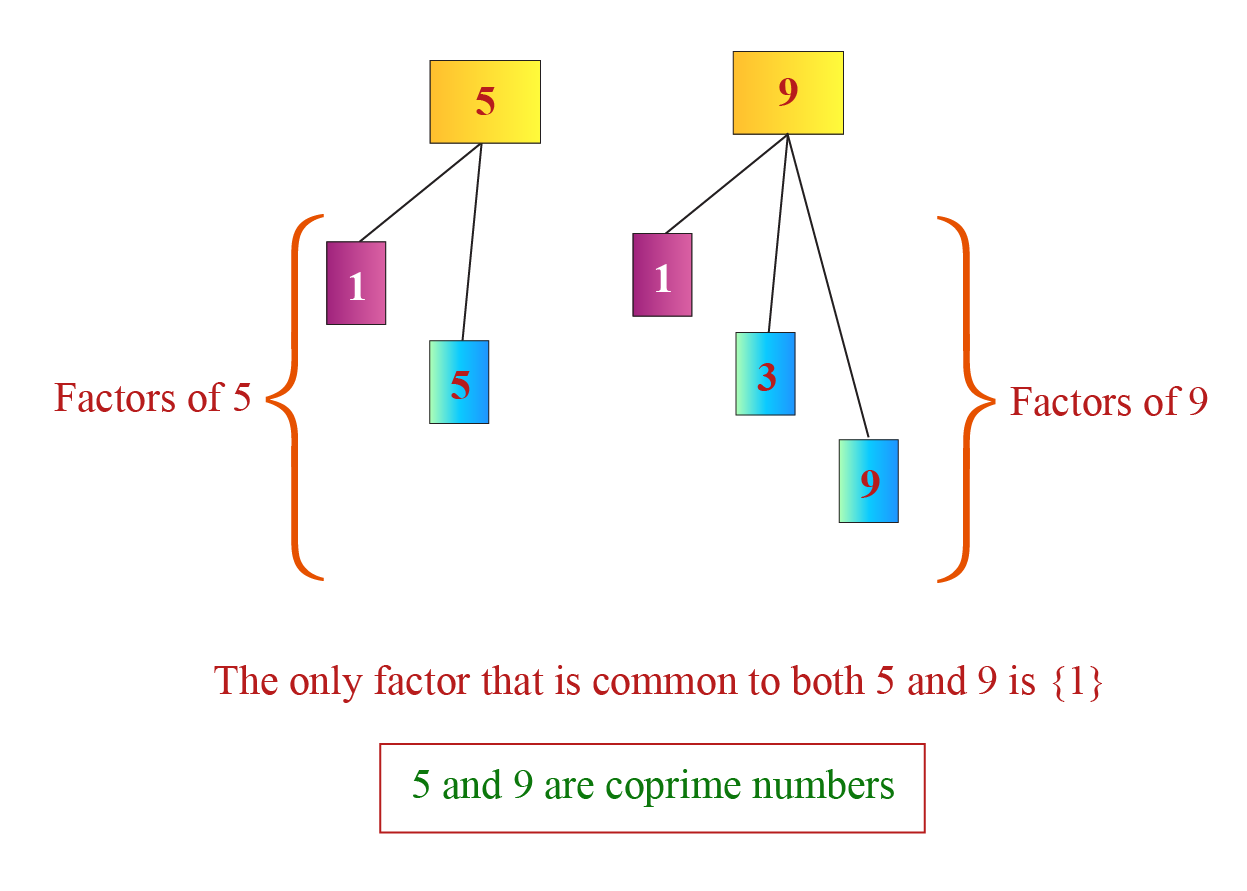Example 2:

$$6$$ and $$10$$ are NOT co-primes. That’s because:

Factors of $$6$$ are $$1,2,3$$ and $$6$$.

Factors of $$10$$ are $$1, 2,5$$ and $$10$$.

Here $$6$$ and $$10$$ have a common factor $$2$$, which is apart from $$1$$, making them NOT coprime.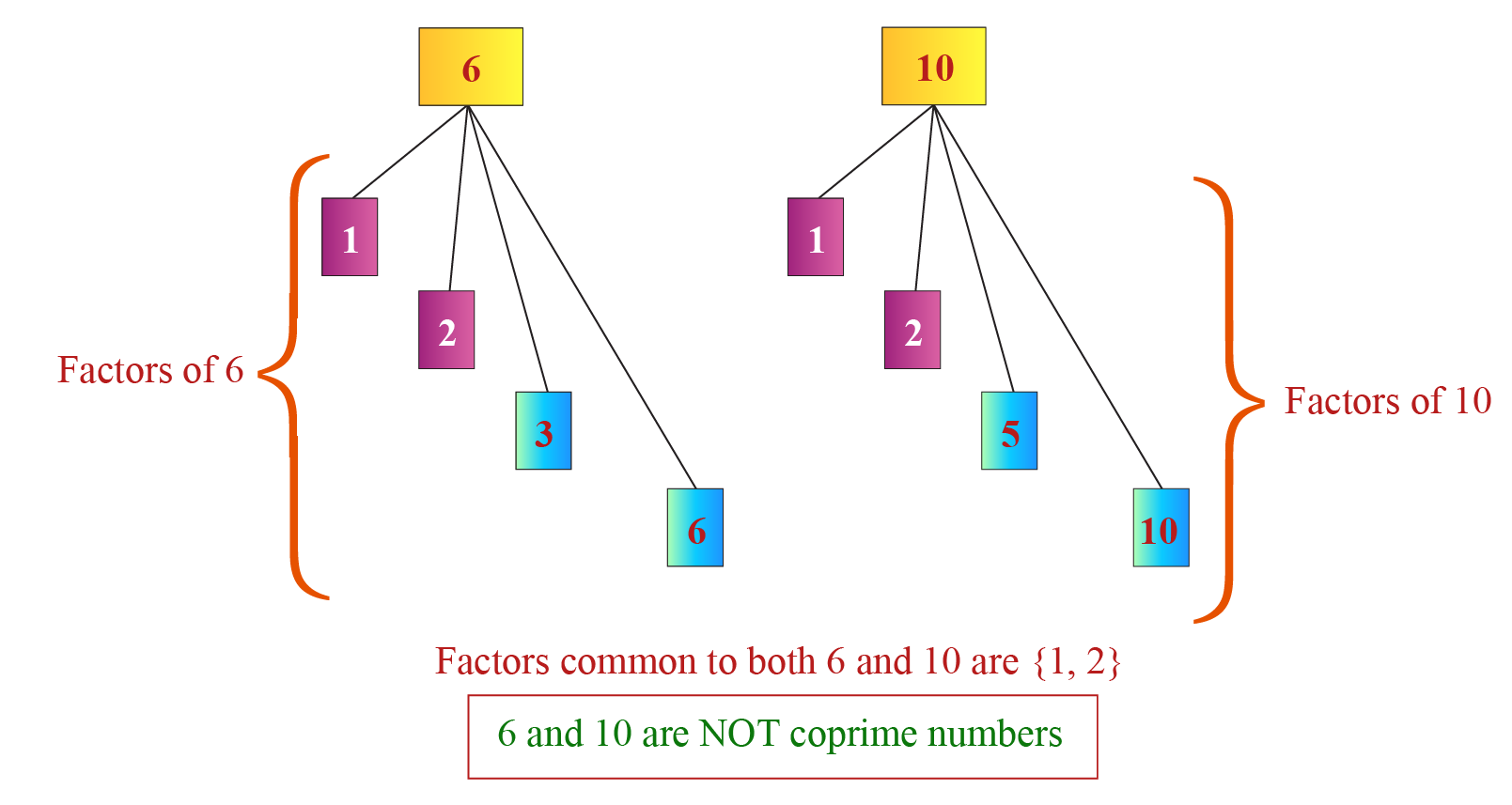Help your child score higher with Cuemath’s proprietary FREE Diagnostic Test. Get access to detailed reports, customised learning plans and a FREE counselling session. Attempt the test now.

## How to Find Prime Numbers?

If you would like to know whether any given number is prime, follow these steps:

• Write all the factors of the given number.
• Count the factors.
• If the number of factors is EXACTLY $$2$$ then the given number is prime.

If the number of factors is more than $$2$$ then the given number is NOT prime (in that case, it is called a composite number).

## Solved Examples

 Example 1

Is $$6$$ prime or NOT?

Solution:

Given number is $$6$$.

The factors of $$6$$ are  $$1, 2, 3, \text{ and }6$$.

Thus $$6$$ has $$4$$ factors.

Since the number of factors of $$6$$ is NOT exactly 2, it is NOT a prime number (so $$6$$ is a composite number).

 ∴ $$6$$ $$is$$ $$NOT$$ $$a$$ $$prime$$ $$number$$.
 Example 2

Is $$37$$ prime or NOT?

Solution:

Given number is $$37$$.

The factors of $$37$$ are $$1 \text{ and } 37$$.

Thus $$37$$ has exactly two factors.

Since the number of factors of $$37$$ is exactly 2, it is a prime number.

 ∴ $$37$$ $$is$$ $$a$$ $$prime$$ $$number$$.
 Example 3

Is $$20$$ prime or NOT?

Solution:

Given number is $$20$$.

The factors of $$20$$ are $$1, 2, 4, 5, 10, \text{ and } 20$$.

Thus $$20$$ has more than two factors.

Since the number of factors of $$20$$ is NOT exactly $$2$$, it is NOT a prime number (So $$20$$ is a composite number).

 ∴ $$20$$ $$is$$ $$NOT$$ $$a$$ $$prime$$ $$number$$.Common Mistakes
1. Mistake: 1 is a prime number.

Fact: A common misconception is to imagine $$1$$ to be a prime number as it has no factors.

$$1$$ is neither prime nor composite.

2. Mistake: Fractions, decimals, and negative integers can be prime numbers

Fact: Since the definition of prime numbers deals with whole numbers that are greater than $$1$$, fractions and decimals that are NOT whole numbers; and the negative integers cannot be prime numbers.

## Prime Numbers Calculator

Let’s enter any whole number here in the box and we will come to know its factors.

We will also come to know whether the number we entered is a prime number.Think Tank
1. Is 53 or 72 a prime number?
2. How many factors does 18 have?
3. Is $$\dfrac{3}{4}$$ a Prime number?
4. How many Prime Numbers are there between 60 and 70?

## Practice Questions

Here are a few activities for you to practice. Select/Type your answer and click the "Check Answer" button to see the result.

## Important Topics

Given below are the list of topics that are closely connected to prime numbers. These topics will also give you a glimpse of how such concepts are covered in Cuemath.

IMO (International Maths Olympiad) is a competitive exam in Mathematics conducted annually for school students. It encourages children to develop their math solving skills from a competition perspective.

## 1. How to find prime numbers?

To find whether a given number is prime, we just find its number of factors. If it’s exactly $$2$$, then the number is prime. You can try out some of the examples given under "Solved Examples" to understand the steps in finding prime numbers.

## 2. What are some examples of prime numbers?

Some examples are $$2, 3, 5, 7, ...$$ etc. You can see the detailed list of prime numbers between $$1$$ to $$100$$ above.

## 3. Are all prime numbers odd?

No. $$2$$ is an even prime number. It is the only even number that is prime.

## 4. What are prime and composite numbers?

A whole number greater than $$1$$ with exactly $$2$$ factors is a prime number and with more than $$2$$ factors is a composite number.

## 5. Why is $$11$$ not a prime number?

$$11$$ is a prime number as it has exactly two factors $$1$$ and $$11$$.

## 6. Why is $$2$$ a prime number?

The number $$2$$ is a prime number because it has exactly two factors, which are $$1 \text{ and } 2$$.

## 7. Can prime numbers be negative?

No, the prime numbers should be only whole numbers greater than $$1$$.

## 8. Which is the largest known prime number?

It is $$2^{82,589,933}$$-1 having $$24,862,048$$ digits.

## 9. What are some tips that can be used while finding prime numbers?

• Any even number other than $$2$$ is NOT a prime number.
• $$2$$ is the only even prime number (it is prime as it has EXACTLY two factors $$1 \text{ and } 2$$).
• Any two-digit number that ends with $$0$$ or $$5$$ is NOT a prime number as any such number is divisible by $$5$$.
• If the sum of the digits of a number (other than $$3$$) is divisible by $$3$$ then the number is NOT a prime number.
• If the difference of the last digit and the number made by the other digits of a number is divisible by $$11$$, then the number is NOT a prime number.
(Because in this case, the number is divisible by $$11$$).

More Important Topics
Numbers
Algebra
Geometry
Measurement
Money
Data
Trigonometry
Calculus